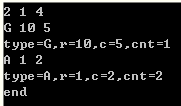`````` #include<iostream>
#include<cstring>
using namespace std;
char p;
int main()
{
//
//freopen("input.txt","r",stdin);
//freopen("output.txt","w",stdout);
//
int n,x,y;
while(scanf("%d%d%d",&n,&x,&y)==3&&n)
{
int r,c,cnt=0;
char type;
for(int i=0;i<n;i++)
{
scanf("%c%d%d",&type,&r,&c);
printf("type=%c,r=%d,c=%d,cnt=%d\n",type,r,c,++cnt);
}
printf("end\n");
}
//printf("n=%d,x=%d,y=%d",n,x,y);
return 0;
}
``````

2空格1空格4回车G空格10空格5回车

type=
,r=-858993460,c=-858993460,cnt=1
type=G,r=10,c=5,cnt=2
end

6个回答

#include
#include

`````` #include<stdio.h>
#include<string.h>
``````

``````#include<iostream>
using namespace std;

int main()
{
int n,x,y;
while(scanf("%d%d%d",&n,&x,&y)==3&&n)
{
int r,c,cnt=0;
char type;
for(int i=0;i<n;i++)
{
cin>>type>>r>>c;
//scanf("%c",&type);
//scanf("%d%d",&r,&c);
printf("type=%c,r=%d,c=%d,cnt=%d\n",type,r,c,++cnt);
}
printf("end\n");
}
system("pause");
return 0;
}
``````lsh_001 不知道是不是我打错了，我之前换成cin是一样的错误，后来我全部重新输入过就好了，cin是可以，谢谢lsh_001 谢谢，不过我之前已经采纳上面的了